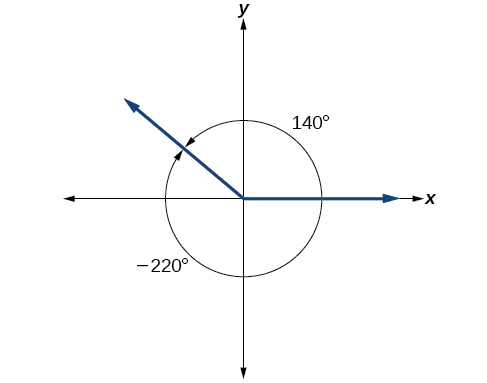# 7.1 Angles  (Page 5/29)

 Page 5 / 29

## Converting degrees to radians

Convert $\text{\hspace{0.17em}}15\text{\hspace{0.17em}}$ degrees to radians.

In this example, we start with degrees and want radians, so we again set up a proportion, but we substitute the given information into a different part of the proportion.

$\begin{array}{ccc}\hfill \frac{\theta }{180}& =& \frac{{\theta }^{R}}{\pi }\hfill \\ \hfill \frac{15}{180}& =& \frac{{\theta }^{R}}{\pi }\hfill \\ \hfill \frac{15\pi }{180}& =& {\theta }^{R}\hfill \\ \hfill \frac{\pi }{12}& =& {\theta }^{R}\hfill \end{array}$

Convert $\text{\hspace{0.17em}}126°\text{\hspace{0.17em}}$ to radians.

$\frac{7\pi }{10}$

## Finding coterminal angles

Converting between degrees and radians can make working with angles easier in some applications. For other applications, we may need another type of conversion. Negative angles and angles greater than a full revolution are more awkward to work with than those in the range of $\text{\hspace{0.17em}}0°\text{\hspace{0.17em}}$ to $\text{\hspace{0.17em}}360°,$ or $\text{\hspace{0.17em}}0\text{\hspace{0.17em}}$ to $\text{\hspace{0.17em}}2\pi .\text{\hspace{0.17em}}$ It would be convenient to replace those out-of-range angles with a corresponding angle within the range of a single revolution.

It is possible for more than one angle to have the same terminal side. Look at [link] . The angle of $\text{\hspace{0.17em}}140°\text{\hspace{0.17em}}$ is a positive angle, measured counterclockwise. The angle of $\text{\hspace{0.17em}}–220°\text{\hspace{0.17em}}$ is a negative angle, measured clockwise. But both angles have the same terminal side. If two angles in standard position have the same terminal side, they are coterminal angles    . Every angle greater than $\text{\hspace{0.17em}}360°\text{\hspace{0.17em}}$ or less than $\text{\hspace{0.17em}}0°\text{\hspace{0.17em}}$ is coterminal with an angle between $\text{\hspace{0.17em}}0°\text{\hspace{0.17em}}$ and $\text{\hspace{0.17em}}360°,$ and it is often more convenient to find the coterminal angle within the range of $\text{\hspace{0.17em}}0°\text{\hspace{0.17em}}$ to $\text{\hspace{0.17em}}360°\text{\hspace{0.17em}}$ than to work with an angle that is outside that range.An angle of   140°   and an angle of   –220°   are coterminal angles.

Any angle has infinitely many coterminal angles because each time we add $\text{\hspace{0.17em}}360°\text{\hspace{0.17em}}$ to that angle—or subtract $\text{\hspace{0.17em}}360°\text{\hspace{0.17em}}$ from it—the resulting value has a terminal side in the same location. For example, $\text{\hspace{0.17em}}\text{100°}\text{\hspace{0.17em}}$ and $\text{\hspace{0.17em}}\text{460°}\text{\hspace{0.17em}}$ are coterminal for this reason, as is $\text{\hspace{0.17em}}-260°.\text{\hspace{0.17em}}$

An angle’s reference angle is the measure of the smallest, positive, acute angle $\text{\hspace{0.17em}}t\text{\hspace{0.17em}}$ formed by the terminal side of the angle $\text{\hspace{0.17em}}t\text{\hspace{0.17em}}$ and the horizontal axis. Thus positive reference angles have terminal sides that lie in the first quadrant and can be used as models for angles in other quadrants. See [link] for examples of reference angles for angles in different quadrants.

## Coterminal and reference angles

Coterminal angles are two angles in standard position that have the same terminal side.

An angle’s reference angle    is the size of the smallest acute angle, $\text{\hspace{0.17em}}{t}^{\prime },$ formed by the terminal side of the angle $\text{\hspace{0.17em}}t\text{\hspace{0.17em}}$ and the horizontal axis.

Given an angle greater than $\text{\hspace{0.17em}}360°,$ find a coterminal angle between $\text{\hspace{0.17em}}0°\text{\hspace{0.17em}}$ and $\text{\hspace{0.17em}}360°$

1. Subtract $\text{\hspace{0.17em}}360°\text{\hspace{0.17em}}$ from the given angle.
2. If the result is still greater than $\text{\hspace{0.17em}}360°,$ subtract $\text{\hspace{0.17em}}360°\text{\hspace{0.17em}}$ again till the result is between $\text{\hspace{0.17em}}0°\text{\hspace{0.17em}}$ and $\text{\hspace{0.17em}}360°.$
3. The resulting angle is coterminal with the original angle.

## Finding an angle coterminal with an angle of measure greater than $\text{\hspace{0.17em}}360°$

Find the least positive angle $\text{\hspace{0.17em}}\theta \text{\hspace{0.17em}}$ that is coterminal with an angle measuring $\text{\hspace{0.17em}}800°,$ where $\text{\hspace{0.17em}}0°\le \theta <360°.$

An angle with measure $\text{\hspace{0.17em}}800°\text{\hspace{0.17em}}$ is coterminal with an angle with measure $\text{\hspace{0.17em}}800-360=440°,$ but $\text{\hspace{0.17em}}440°\text{\hspace{0.17em}}$ is still greater than $\text{\hspace{0.17em}}360°,$ so we subtract $\text{\hspace{0.17em}}360°\text{\hspace{0.17em}}$ again to find another coterminal angle: $\text{\hspace{0.17em}}440-360=80°.$

The angle $\text{\hspace{0.17em}}\theta =80°\text{\hspace{0.17em}}$ is coterminal with $\text{\hspace{0.17em}}800°.\text{\hspace{0.17em}}$ To put it another way, $\text{\hspace{0.17em}}800°\text{\hspace{0.17em}}$ equals $\text{\hspace{0.17em}}80°\text{\hspace{0.17em}}$ plus two full rotations, as shown in [link] .

#### Questions & Answers

x exposant 4 + 4 x exposant 3 + 8 exposant 2 + 4 x + 1 = 0
x exposent4+4x exposent3+8x exposent2+4x+1=0
HERVE
How can I solve for a domain and a codomains in a given function?
ranges
EDWIN
Thank you I mean range sir.
Oliver
proof for set theory
don't you know?
Inkoom
find to nearest one decimal place of centimeter the length of an arc of circle of radius length 12.5cm and subtending of centeral angle 1.6rad
factoring polynomial
find general solution of the Tanx=-1/root3,secx=2/root3
find general solution of the following equation
Nani
the value of 2 sin square 60 Cos 60
0.75
Lynne
0.75
Inkoom
when can I use sin, cos tan in a giving question
depending on the question
Nicholas
I am a carpenter and I have to cut and assemble a conventional roof line for a new home. The dimensions are: width 30'6" length 40'6". I want a 6 and 12 pitch. The roof is a full hip construction. Give me the L,W and height of rafters for the hip, hip jacks also the length of common jacks.
John
I want to learn the calculations
where can I get indices
I need matrices
Nasasira
hi
Raihany
Hi
Solomon
need help
Raihany
maybe provide us videos
Nasasira
Raihany
Hello
Cromwell
a
Amie
What do you mean by a
Cromwell
nothing. I accidentally press it
Amie
you guys know any app with matrices?
Khay
Ok
Cromwell
Solve the x? x=18+(24-3)=72
x-39=72 x=111
Suraj
Solve the formula for the indicated variable P=b+4a+2c, for b
Need help with this question please
b=-4ac-2c+P
Denisse
b=p-4a-2c
Suddhen
b= p - 4a - 2c
Snr
p=2(2a+C)+b
Suraj
b=p-2(2a+c)
Tapiwa
P=4a+b+2C
COLEMAN
b=P-4a-2c
COLEMAN
like Deadra, show me the step by step order of operation to alive for b
John
A laser rangefinder is locked on a comet approaching Earth. The distance g(x), in kilometers, of the comet after x days, for x in the interval 0 to 30 days, is given by g(x)=250,000csc(π30x). Graph g(x) on the interval [0, 35]. Evaluate g(5)  and interpret the information. What is the minimum distance between the comet and Earth? When does this occur? To which constant in the equation does this correspond? Find and discuss the meaning of any vertical asymptotes.
The sequence is {1,-1,1-1.....} hasByByBy Jordon HumphreysBy Sam LuongByBy